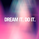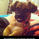צפיות ‎28072‎
John Carter's TTM Squeeze indicator
```// create base on http://www.dailyfx.com/forex_forum/coding-strategy-advisors-indicators-functions/237563-ttm-squeeze-indicator.html
study("TTM Squeeze")
length = input(title="Length", type=integer, defval=20, minval=0)
bband(length, mult) =>
sma(close, length) + mult * stdev(close, length)
keltner(length, mult) =>
ema(close, length) + mult * ema(tr, length)

e1 = (highest(high, length) + lowest(low, length)) / 2 + sma(close, length)
osc = linreg(close - e1 / 2, length, 0)
diff = bband(length, 2) - keltner(length, 1)
osc_color = osc < osc ? osc >= 0 ? #00ffff : #cc00cc : osc >= 0 ? #009b9b : #ff9bff
mid_color = diff >= 0 ? green : red

plot(osc, color=osc_color, style=histogram, linewidth=2)
plot(0, color=mid_color, style=circles, linewidth=3)
```

## תגובותHi Jonh: could you please some time to explain basics readings of your indicator. I´ve seen few respected traders using it. Thank you so much before hand !!!